This section is Section 2 of 3.

Speed as well as accuracy is important in this section. Work quickly, or you might not finish the paper. There are no  penalties for incorrect responses, only marks for correct answers, so you should attempt all 27 questions. Each question is worth one mark.

You must complete the answers within the time limit. Calculators are NOT permitted.

Good Luck!

Note – if press “End Exam” you can access full worked solutions for all past paper questions

The following statements relate to the flow of blood through the heart.

1 Oxygenated blood flows through the right side of the heart.

2 The vena cava empties deoxygenated blood into the ventricle.

3 The heart pumps oxygenated blood through the pulmonary artery.

4 There is a valve that prevents backflow of blood from the aorta into the left ventricle.

1. Which of these statements is/ are correct?
0
0

## Explanation

Statement 1 is incorrect – deoxygenated blood flows through the right side of the heart.

Statement 2 is incorrect because blood from the vena cava enters the atrium.

Statement 3 is incorrect because the pulmonary artery is one of the few arteries that carries deoxygenated blood.

Statement 4 is correct because there are valves in the base of the aorta to prevent the backflow of blood into the ventricle, when the ventricle relaxes.

Post Comment

1 CuSO₄ + Zn → Cu + ZnSO₄

2 HCl + NaOH → NaCl +H₂O

3 Mg + H₂SO₄ → MgSO₄ + H₂

4 Pb(NO₃)₂ + 2Kl → Pbl₂ + 2KNO₃

2. Which of the following reactions are redox reactions?
0
0

## Explanation

Reaction 1 is a redox reaction because there are changes in oxidation states (ionic charges): the oxidation state of copper changes from +2 to 0 (Cu2+ + 2e– → Cu [reduction]), and zinc changes from 0 to +2 (Zn → Zn2+ + 2e– [oxidation]).

Reaction 2 is not a redox reaction because there is no electron transfer (no change in ionic charge, nor oxidation state).

Reaction 3 is a redox reaction because there are changes in oxidation states (ionic charges): the oxidation state of magnesium changes from 0 to +2 (Mg → Mg2+ + 2e– [oxidation]), and hydrogen changes from +1 to 0 (2H+ + 2e– → H2 [reduction]).

Reaction 4 is not a redox reaction because there is no transfer of electrons.

Post Comment

1 Microwaves have a shorter wavelength than all other electromagnetic waves.

2 For identical amplitudes, waves with the largest wavelength transfer the most energy.

3 The speed of electromagnetic waves is inversely proportional to their frequency.

4 Ultraviolet radiation can cause cataracts.

3. Which of these statements is/ are correct?
1
1

## Explanation

Statement 1 is incorrect; microwaves have a longer wavelength than infrared radiation and many other parts of the electromagnetic spectrum.

Statement 2 is incorrect; energy increases with frequency (and consequently decreases with wavelength), and so in fact the shortest wavelengths transfer the most energy.

Statement 3 is incorrect; the wavelength is inversely proportional to frequency, and the speed is the constant for this relationship ( u =  f × λ).

Statement 4 is correct; ultraviolet waves are higher frequency than visible light, so carry more energy and can damage corneas, causing cataracts.

Post Comment

Simplify: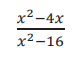4.
0
0

## Explanation

Factorise both numerator and denominator.

Numerator: x is the common factor, hence:

x2 − 4x = x (x − 4)

Denominator: Via difference of two squares,x2− 16 = (x + 4) (x − 4)

Cancel both (x − 4) , as they appear in both numerator and denominator: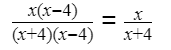Post Comment

Before a cell can divide by mitosis, DNA synthesis has to take place. Following DNA synthesis, the DNA is separated into each half of the cell and then the cell divides.

The graph below shows the DNA content per cell over a period of time.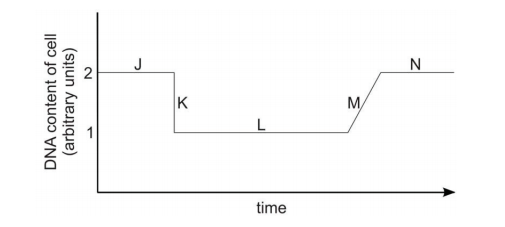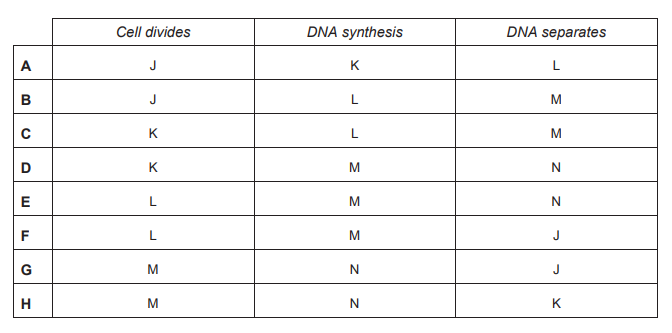5. Which of the letters on the graph represent the sequence of the three events described above?
0
0

## Explanation

DNA synthesis doubles the DNA content in the cell, so the content will increase from 1 to 2 arbitrary units, which is M. The DNA separates within the cell. However, the total content is still 2, hence this is N or J. The cell divides in half, which halves the DNA content per cell back to 1, which is K.

Post Comment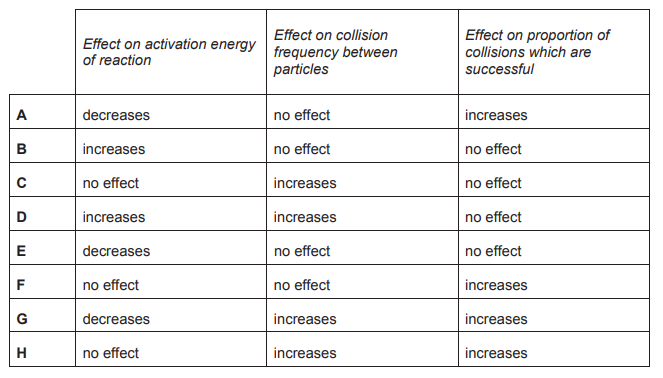6. Which row in the table correctly explains why an increase in temperature increases the rate of a reaction?
0
0

## Explanation

When the temperature of a reaction is increased, the particles gain kinetic energy so they move faster and increase the frequency of collisions. The proportion of successful collisions will also increase because the proportion of particles with energy greater than the activation energy has increased. This is why a small increase in temperature results in a large increase in the rate of a reaction. The activation energy is the minimum energy required by colliding particles to result in a successful collision and, therefore, a chemical change. Activation energy is a measure of the energy required to break chemical bonds and is not affected by temperature. Hence, an increase in temperature will have no effect on the activation energy but it will increase the frequency of collisions as well as the proportion of successful collisions.

Post Comment

Which one of the following is a unit of current?

7.
0
0

## Explanation

From power: power (watts) = current (amps) x voltage (volts)

current (amps)=power (watts)/voltage (volts) , so unit (ampere) = watt/volt

Post Comment

Given that 4p x 8q = 2n ,express n in terms of p and q.

8.
0
0

## Explanation

Express 4 and 8 as powers of 2: 4 =  ,=

Hence: 4p x 8q= (2²)p x (2³)q = p x q = p+³q

Hence, n = 2p + 3q

Post Comment

Insulin is a protein involved in the regulation of human blood glucose levels.

Genetic engineering can be used to allow the large-scale production of human insulin.

9. Which statement describes the process of genetic engineering in this case?
0
0

## Explanation

Post Comment

Methanol can be oxidised by hydrogen peroxide to produce carbon dioxide and water.

aCH₃OH + 3H₂O₂ → CO₂ + bH₂O

10. What is the value of b when this equation is balanced?
0
0

## Explanation

When balancing this chemical equation, you must have the same number of carbon atoms, hydrogen atoms and oxygen atoms on both the reactant side and the product side of the equation. This is only true for the correct answer, C. ‘a’ can be calculated first. With just one carbon on the right hand side of the equation it can be deduced that a=1. With this knowledge, the remainder of the equation can be balanced and ‘b’ found. For instance, recognising 7 oxygen atoms are present on the left, and therefore 7 oxygen atoms much be present on the right, means that b must be 5.

Post Comment

Two rods, X and Y, are made from different electrically insulating materials. A student rubs rod X, which is initially uncharged, with a cloth, then holds it near to rod Y. The two rods repel each other.

11. Which statement explains why repulsion occurs in this experiment?
0
0

## Explanation

Only electrons are transferred when an object becomes charged. Hence options C, D, G and H are all incorrect. The object becomes negatively charged if it gains electrons and positively charged if it loses electrons. For repulsion to occur, two objects must have like charges, so either rod X gained electrons from the cloth and rod Y was negatively charged (which is not one of the options) or rod X lost electrons to the cloth and rod Y was positively charged, option F.

Post Comment

In a town, the bearing of the library from the station is x°.

The theatre is due east of the library.

The theatre and the station are equidistant from the library.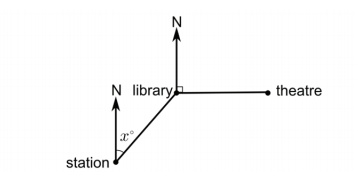12. What is the bearing (in degrees) of the station from the theatre?
0
0

## Explanation

Extend north line down at L and recall that alternate angles between parallel lines are equal, so angle SLT = 90 + x

Because the interior angles of any triangle add up to 180°, LTS + LST = 180 − (90 + x)

LTS + LST = 90 − x.

Triangle SLT is isosceles so both angles LTS and LST = (90−x)/2

Bearing S from T = 270 – ( (90−x)/2) = 270 − 45 + x/2 = 225 + x/2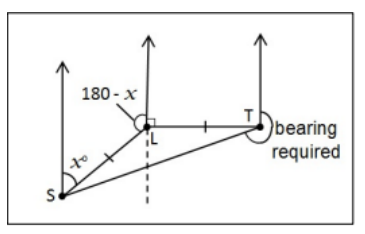Post Comment

In humans, the water content in the blood is regulated via the hormonal system.

1 pituitary gland releases less ADH

2 pituitary gland releases more ADH

3 increase in water reabsorption by the kidneys

4 decrease in water reabsorption by the kidneys

5 increased reabsorption of glucose in the kidneys

6 decreased reabsorption of glucose in the kidneys

13. Which of the following occur when the water content of the blood is too low?
0
0

## Explanation

The water content in the blood is detected by the brain, which controls how much ADH is released by the pituitary gland. When the water content is too low, the pituitary gland releases more ADH. This causes an influx in water reabsorption by the kidneys, hence a high volume of water passes into the blood.

Post Comment

Which of the following statements about but-1-ene are true?

1 it can form a polymer

2 it contains CC single bonds only

3 it conforms to the general formula CnH₂n

4 it decolourises bromine water

5 it is saturated

14.
0
0

## Explanation

But-1-ene is an alkene, and alkenes can form addition polymers, so statement 1 is true.

But-1-ene contains 1 C=C (carbon-carbon double bond), so statement 2 is false.

Alkenes have twice as many hydrogen atoms as carbon atoms, so they, and hence but-1-ene, conform to the general formula CnH2n, so statement 3 is true.

Alkenes decolourise bromine water, so statement 4 is true.

But-1-ene contains 1 C=C bond, so is unsaturated, so statement 5 is false.

Therefore, only statements 1, 3 and 4 are true.

Post Comment

A container is filled with water at 20 °C and placed in a room that is also at 20 °C. The container can be fitted with an internal electric cooling unit, in one of the three labelled positions P, Q or R. The outside of the container can either be painted dull black, or be covered in shiny aluminium foil.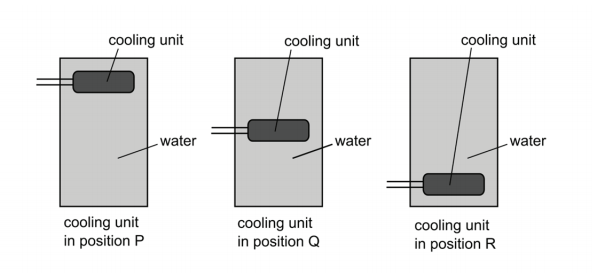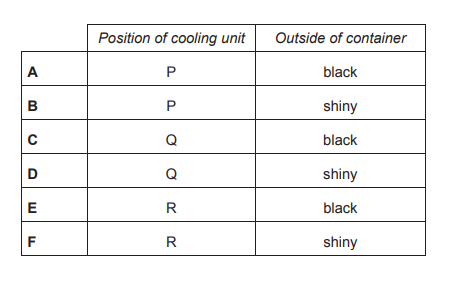15. In order to cool all the water as quickly as possible to 5 °C, in which position should the cooling unit be fitted, and should the outside of the container be dull black or shiny?
0
0

## Explanation

Cooled water will become more dense and fall, causing a convection current in the container. The cooling unit should be positioned at the top to ensure that all the water is cooled, including the warmer, less dense water at the top. The water starts at room temperature, and will soon become cooler than its surroundings. This means that a dark surface would absorb more infra-red from the surroundings than it would emit. A shiny surface reduces this effect and will help to keep the water cooler than the surroundings.

Post Comment

Three classes in a school all took the same test. Class 1 achieved a mean score of 61, Class 2 achieved a mean score of 63, and class 3 achieved a mean score of 70. The mean score of the students for all three classes combined was 65. Class 1 contains twice as many students as Class 2.

16. Which one of the following statements about the number of students in class 3 is true?
0
2

## Explanation

Class 1 contains twice as many students as Class 2 – if there are n students in Class 2, then there are 2n students in Class 1. The total of all of the scores in Class 1 must be 61 × 2n = 122n. The total of all of the scores in Class 2 must be 63n. If there are m students in Class 3, then the total of all the scores in Class 3 must be 70m. The total score of all of the students must be 65 × (2n + n + m), so 195n + 65m = 122n + 63n + 70m. This simplifies to 10n = 5m, so m = 2n. The number of students in Class 3 is 2n, which is the same as the number of students in Class 1.

Post Comment

1 Emulsification by bile makes smaller lipid droplets, each with a smaller surface area.

2 Bile contains an alkali to reduce the pH of the material from the stomach.

3 Lipase secreted in bile breaks bonds in lipids to produce glycerol and fatty acids.

17. Which of the following statements about lipid digestion in the small intestine is /are correct?
3
0

## Explanation

Emulsification is the breakdown of large lipid globules into smaller droplets by the action of bile salts. Each single droplet has a smaller surface area than a single large droplet, but collectively the many more smaller droplets formed have a higher surface area to volume ratio than fewer large droplets. Therefore statement 1 is correct.

Bile is alkalic, but this increases the pH of the material from the stomach rather than decreasing it, therefore statement 2 is incorrect.

Lipase does hydrolyse bonds in lipids to produce glycerol and fatty acids, but lipase is not secreted in bile, it is secreted from the pancreas into pancreatic fluid, which enters the small intestine via the pancreatic duct. So statement 3 is also incorrect.

Hence, the only correct statement is 1.

Post Commentyageen Medicmind Tutor

Wed, 06 Oct 2021 09:46:46

bile emulsifies large lipid molecules to smaller droplets which have a higher surface area ( smaller volume), making it easier for lipase to break them downs Medicmind Tutor

Mon, 27 Jun 2022 14:02:55

yeah you are right 1 is wrong as the smaller droplets have higher surface area so that lipase from pancrease can work more efficientlys Medicmind Tutor

Mon, 27 Jun 2022 14:09:20

sorry to anyone reading question 1 and not understanding it it says "each" which makes the statement currect in total the emulsification increases the surface area but each droplet has less surface area than the large droplets they originated from. hope it helps.

An organic compound is found to contain 6 parts of carbon, 1 part of hydrogen and 8 parts of oxygen by mass.

6 g of a gaseous sample of the compound would have a volume of 2.4 dm3 at room temperature and pressure.

(Ar: H = 1; C = 12; O = 16)

(1 mole of any gas occupies 24 dm³ at room temperature and pressure)

18. Which formula (A–E) is the molecular formula for this compound?
0
1

## Explanation

You need to find: the molecular mass, from the mass, volume and mole information, and then dividing this mass over the 3 elements to figure out how many atoms of each are present in the molecule.

Using the molar gas volume given (1 mole = 24dm3 ), we can deduce that 2.4 dm3 of the compound must contain 0.1 moles.

Hence, 0.1 moles of the compound weighs 6g. So, 1 mole of the compound would weigh 60g.

Hence the relative molecular mass must be 60.

The ratio of C : H : O by mass is 6 : 1 : 8; dividing the mass into this ratio gives us the mass of each element in the molecule. C: 6 15 × 60 H: 1 15 × 60 O: 8 15 × 60

The relative masses are: C: 24 H: 4 O: 32 Using the Ar values, we can see this relates to 2 carbons, 4 hydrogens, and 2 oxygens. The molecular formula is C2H4O2.

Post Comment

The displacement / time graph shown represents a wave of wavelength 1.5 cm.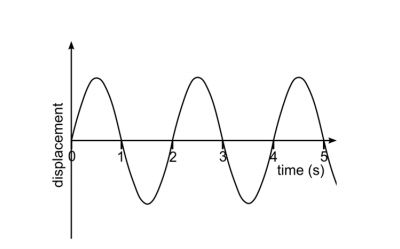19. What is the speed of the wave?
1
0

## Explanation

Period= 2s hence, frequency =(1/ 2) = 0.5 Hz u f × λ = 0.50 × 1.5 = 0.75cm/s

Post Comment

The diagram shows part of a glass structure. PQRS is a horizontal square with sides of 1 metre, and point X is 4 metres vertically above P.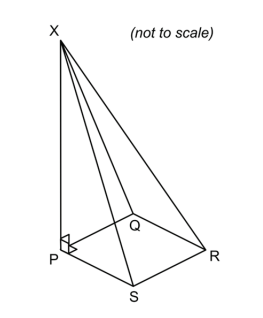20. What is the cosine of the angle that XR makes with the horizontal?
0
0

## Explanation

The angle required is XRP. Using the Pythagorean Theorem PR = √2, since PR is a diagonal of square PQRS. Then triangle XPR is a right angled triangle as X is vertically above P, and by Pythagoras XR = √18 = 3√2

Hence, cos(XRP) = PR/XR = √2/(3√2) = 1/3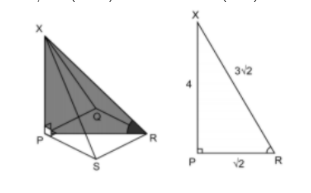Post Comment

In order to function, the cells of the brain need large amounts of energy. Any reduction in the function of these cells can have serious consequences for the body. For example, a loss of oxygen supply to the brain can cause unconsciousness within 5–10 seconds.

1 Neurons in the brain are capable of relying on anaerobic respiration for long periods of time.

2 The homeostatic systems of the body will constantly have to work to resist temperature increases in the brain.

3 An overdose of insulin in the body could produce a serious loss of brain function, such as inducing a coma.

4 During normal functioning of neurons in the brain, high levels of carbon dioxide could be produced.

21. Which of the following statements are true?
1
0

## Explanation

Statement 1 is not true. In the opening paragraph, it is stated that oxygen supply is essential for neurons in the brain to function for more than 5 – 10 seconds. Hence, anaerobic respiration cannot be used for prolonged periods of time.

Statement 2 is true because a by-product of aerobic respiration is heat so as respiration occurs, the body will have to work to counteract the corresponding increase in temperature.

Statement 3 is true – too much insulin will lower body glucose levels and this could prevent sufficient respiration from occurring, resulting in a lack of energy and therefore brain function.

Statement 4 is true because carbon dioxide is produced during aerobic respiration.

Post Comment

Graphene is a new material composed of carbon atoms arranged in tightly bound hexagons just one atom thick.

The diagram shows a simplified structure of graphene.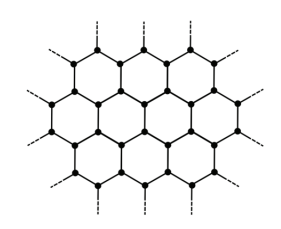1 high melting point

2 good electrical conductivity

3 soluble in water

22. Considering its structure, which of the properties below could be predicted about graphene?
0
0

## Explanation

The graphene description indicates that it has a giant covalent structure. Typically, substances with a giant covalent structure have high melting and boiling points; they do not dissolve in water and they do not conduct electricity. However, the structure of graphene is very similar to that of graphite in that each C atom is covalently bonded to only 3 other C atoms which means that each C atom has a ‘free’ electron. This ‘free’ electron can migrate within the structure, so enabling graphene to conduct electricity. Graphene should have a high melting point and good electrical conductivity but not dissolve in water.

Post Comment

When a particular nucleus of uranium-235 undergoes the process of nuclear fission, it absorbs a neutron and then splits into a nucleus of barium and a nucleus of krypton, as well as releasing further neutrons.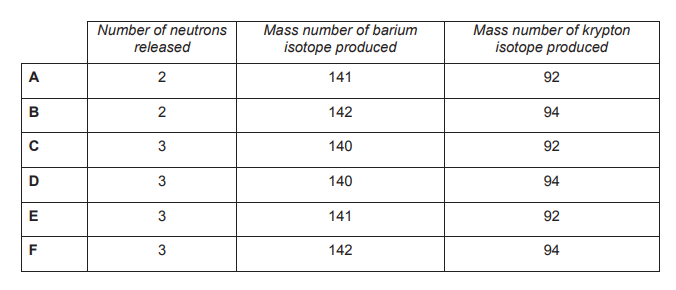23. Which one of the rows of the table below gives the correct number of neutrons released and the isotopes of barium and krypton produced by this nuclear reaction?
0
0

## Explanation

The total mass number of the particles before fission is 235 + 1 = 236 (uranium + neutron). As the total mass number is conserved, the total mass number of the particles after fission must also be 236. E) is the only statement in which the products have a total mass of 236 units.

A) has products with a total mass of 235 units.

B) has products with a total mass of 238 units.

C) has products with a total mass of 235 units.

D) has products with a total mass of 237 units.

F) has products with a total mass of 239 units.

Post Comment

A test is developed to detect a certain medical condition. The test is not perfect, and sometimes gives incorrect results. The behaviour of the test on 1000 randomly selected members of the population is shown in this tree diagram, where the following notation is used:

• C = has the condition
• C′ = does not have the condition
• T = tests positive for the condition
• T′ = tests negative for the condition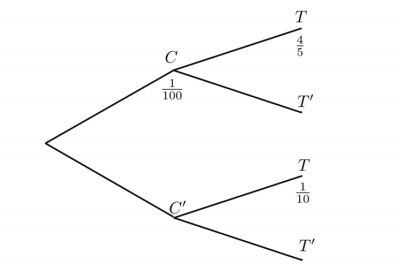Three of the branches’ proportions are shown in the tree diagram:

1 / 100 of the 1000 people have the condition

4 / 5 of those with the condition test positive for the condition

1 / 10 of those without the condition test positive for the condition

A person is selected at random from these 1000 people, and tests positive for the condition.

24. What is the probability that this person has the condition?
0
0

## Explanation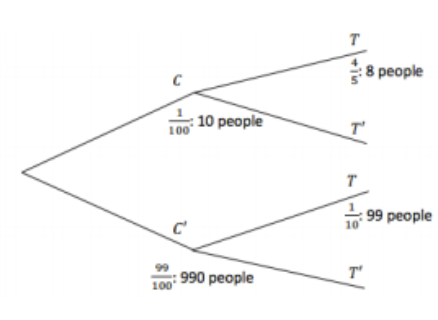Hence, 8 + 99 = 107 people test positive, of which 8 have the condition, so the probability that someone has the condition, given that they test positive, is 8/107.

This is only about 7.5%, so the test is quite poor on this population: over 90% of the positive test results are false positives. Tests for rare conditions which are applied indiscriminately will often suffer from this problem, and can lead to costly treatments (physically, financially and emotionally) for many perfectly healthy people.

Post Comment

A woman has a recessive genetic condition but neither of her parents has the condition.

25. Which one of the following could not be true?
0
0

## Explanation

In order to have the condition, the woman must have 2 copies of the recessive allele, as stated in the question. In order to inherit 2 copies, she must have inherited a recessive copy from each of her parents. As neither of them have the disorder, they must be heterozygous, hence A is true. Her parents must have inherited a recessive allele each from their parents (the woman’s grandparents) so they could all be carriers (D), one of each set of grandparents could be a carrier (C) or they may have the condition (B). In order for the woman to develop a condition that requires two copies of the recessive allele, however, the DNA mutation in one gamete is not sufficient. DNA in the gametes from both parents would have had to mutate if neither of them possessed the recessive allele to start with. Therefore E cannot be true.

Post Comment

Which of the following atoms / ions contain(s) exactly 18 electrons?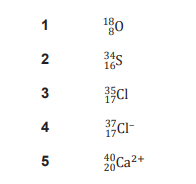26.
0
0

## Explanation

The number of electrons is equal to the number of protons for a neutral atom. For a negative ion, add on the magnitude of the ion’s charge to the proton number to calculate the number of electrons it has, and for a positive ion subtract the magnitude of the charge of the ion from the proton number of the element. O has 8 electrons, S has 16 electrons, Cl has 17 electrons, Cl- has 17+1=18 electrons and Ca2+ has 20-2=18 electrons. Therefore only Cl- (4) and Ca2+ (5) have 18 electrons.

Post Comment

The graph shows the variation with time of the height through which a crane lifts a mass of 20 kg.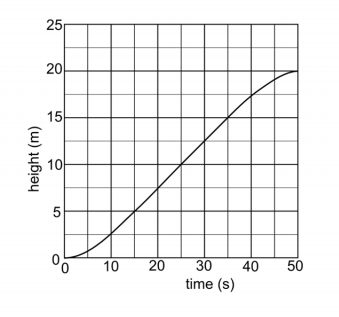Assume the gravitational field strength g is 10 N /kg, and that the effects of air resistance and friction are negligible.

27. What is the power output of the crane when the mass is at a height of 10 m?
0
2

## Explanation

Power output in this question is about the change in potential energy (mgh) per unit time. The mass and gravitational field strength are constant, so the change in potential energy with time is directly related to the change in height with time. In the graph we can see that the change in height with time (the gradient) is constant from 15s to 35s (which includes the time at which it is at the height of 10 m). Hence, we can use this as the time interval from which to calculate the power and energy change. The change in height is 10 m (15–5m) in this interval of 20s. This relates to a change in potential energy of 2000 J (mgh = 20×10×10) in the interval. The power output is given by change in potential energy, divided by the time interval: 2000/20 = 100W.

Post CommentGuest Medicmind Tutor

Thu, 21 Oct 2021 18:10:55

Why use a time interval when an exact time (10 seconds) is stated?Guest Medicmind Tutor

Thu, 21 Oct 2021 18:11:48

I meant 15 seconds, which is shown on the graph at 10m.Guest Medicmind Tutor

Fri, 22 Oct 2021 23:41:00

I think it's so that the gradient is constant(from 15s to 35s it is constant)s Medicmind Tutor

Mon, 27 Jun 2022 14:20:41

bruh its 25 seconds at h=10

## Instructions

Below is a summary of your answers. You can review your questions in three (3) different ways.

The buttons in the lower right-hand corner correspond to these choices:

2. Review questions that are incomplete.
3. Review questions that are flagged for review. (Click the 'flag' icon to change the flag for review status.)

You may also click on a question number to link directly to its location in the exam.

## Instructions

This review section allows you to view the answers you made and see whether they were correct or not. Each question accessed from this screen has an 'Explain Answer' button in the top left hand side. By clicking on this you will obtain an explanation as to the correct answer.

At the bottom of this screen you can choose to 'Review All' answers, 'Review Incorrect' answers or 'Review Flagged' answers. Alternatively you can go to specific questions by opening up any of the sub-tests below.

x

## When should we call you?

It would be great to have a 15m chat to discuss a personalised plan and answer any questions

## What time works best for you? (UK Time)

Pick a time-slot that works best for you ?

Submit

Submit